# C++ Program to Delete an Element from an Array

In this article, you will learn how to delete an element from an array in C++ and get the code for doing so. The program is created in the following ways:

## In C++, delete an element from an array.

To delete an element from an array in C++ programming, you have to ask the user to enter the array's 10 elements first. And then ask for the element that has to be deleted. Now search for that number or element and delete it if found. Otherwise, print a message such as "Element not found."

The question is, "Write a program in C++ that deletes an element from an array." Here is the answer to this question:

```#include<iostream>
using namespace std;
int main()
{
int arr, tot=10, i, elem, j, found=0;
cout<<"Enter 10 Array Elements: ";
for(i=0; i<tot; i++)
cin>>arr[i];
cout<<"\nEnter Element to Delete: ";
cin>>elem;
for(i=0; i<tot; i++)
{
if(arr[i]==elem)
{
for(j=i; j<(tot-1); j++)
arr[j] = arr[j+1];
found++;
i--;
tot--;
}
}
if(found==0)
cout<<"\nElement doesn't found in the Array!";
else
cout<<"\nElement Deleted Successfully!";
cout<<endl;
return 0;
}```

This program was built and runs under the Code::Blocks IDE. Here is its sample run: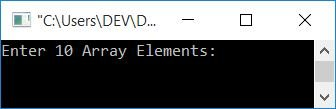Now supply any 10 numbers, say 1, 2, 3, 4, 5, 6, 7, 8, 9, and 10, as 10 array elements. Enter the number or element, say 3, that must be deleted from the list after entering 10 elements for the array arr[]. Here is the final snapshot of the sample run: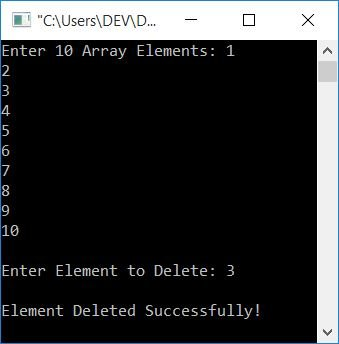Note: If the entered element (that has to be deleted) is found in repeated order (more than once), then the element gets deleted from all the positions. That is, repeated elements also get deleted.

When the user enters 10 array elements as 1, 2, 3, 4, 5, 6, 7, 8, 9, 10, then it gets stored in the array arr[] in the following way:

• arr=1
• arr=2
• arr=3
• arr=4
• arr=5
• arr=6
• arr=7
• arr=8
• arr=9
• arr=10

And the entered element, say 3, that has to be deleted, gets stored in elem. Now the dry run (inside the for loop) of the above program goes like this:

• Initial value, found=0, tot=10
• At the first evaluation of the for loop, 0 gets initialized to i. The initialization (of the for loop) happens first and only once. Now i=0
• The condition i<tot or 0<10 evaluates to be true, therefore program flow goes inside the loop.
• And using if, the condition arr[i]==elem or arr==3 or 1==3 evaluates to be false; therefore, program flow does not go inside if's body, but rather to the updating part of the for loop and increments the value of i. Now i=1
• And again, the condition i<tot or 1<10 evaluates to be true; therefore, the program flow again goes inside the loop and evaluates the condition of the if block.
• And again, the condition arr[i]==elem or arr==elem or 2==3 evaluates to be false; therefore, program flow again increments i. Now i=2
• Because the condition of the for loop evaluates to true the third time, the program flow again goes inside the loop at this time.
• But at this time, the condition of "if" evaluates to be true because element at index number 2 is 3, which equals the value stored in elem (the element that has to be deleted).
• In other words, the condition arr[i]==elem, arr==3, or 3==3 evaluates to true.
• Therefore, program flow goes inside the if's body, and executes all of its statements.
• That is, using for This (current or second) index's elements are all shifted back one index.
• That is, at first, the value of i (2) gets initialized to j. So j=2
• And the condition j<(tot-1) or 2<(10-1) or 2<9 evaluates to be true, therefore program flow goes inside the loop, and arr[j+1] or arr[2+1] or arr or 4 (value at index number 3) gets initialized to arr[j] or arr
• Now the value of j gets incremented. So j=3
• Again, the condition j<(tot-1) or 3<(10-1) or 3<9 evaluates to true, so program flow returns to the loop, and arr[j+1] or arr[3+1] or arr or 5 is initialized to arr[j] or arr.
• This procedure is repeated until the condition j<(tot-1) evaluates to false.
• When the condition evaluates to false, the loop's second evaluation is terminated for the time being.
• And the value of found gets incremented. Its value is initialized with 0 at the start of the program to check whether it contains its value (0) or not, after evaluating both for loops. If it has a value of 0, the program flow will never enter the if block. That means no element from the array gets matched to the element entered as an element to be deleted. And if its value is not 0, then the element gets matched and deleted from that array.
• Don't forget to decrement the value of i by one to start from the index where we came here in the if block to compare with the new element that has shifted to the index from where the element was previously deleted.
• And the value of tot gets decremented, because one element gets deleted, so the size of array becomes 1 less than before.
• Now the value of i gets incremented, and again the condition of the outer for loop gets evaluated.
• This process continues until the condition of the outer for loop evaluates to be false.
• In this way, the element (along with the repeated one) gets deleted from an array.
• After the loop's execution is complete, simply check the value of found and print the message accordingly.

Note: In the above program, if an element is found at any index, say 2, in the array, then from here, all the elements get moved one index back. That is, an element from the third index is moved to the second, an element from the fourth index is moved to the third, and so on.

### Delete an element and print a new array

This program has two extra features compared with the previous one. The two extra features are:

• Allows the user to specify the array's size.
• prints the new array after deleting the element.

The question is: write a program in C++ that deletes an element and prints the new array. The answer to this question is given below:

```#include<iostream>
using namespace std;
int main()
{
int arr, tot, i, elem, j, found=0;
cout<<"Enter the Size: ";
cin>>tot;
cout<<"Enter "<<tot<<" Array Elements: ";
for(i=0; i<tot; i++)
cin>>arr[i];
cout<<"\nEnter Element to Delete: ";
cin>>elem;
for(i=0; i<tot; i++)
{
if(arr[i]==elem)
{
for(j=i; j<(tot-1); j++)
arr[j] = arr[j+1];
found=1;
i--;
tot--;
}
}
if(found==0)
cout<<"\nElement doesn't found in the Array!";
else
{
cout<<"\nElement Deleted Successfully!";
cout<<"\n\nThe New Array is:\n";
for(i=0; i<tot; i++)
cout<<arr[i]<<"  ";
}
cout<<endl;
return 0;
}```

Here is its sample run with user input.

• 8 in size
• 1, 2, 3, 4, 2, 5, 2 as 8 array elements
• 2 as an element to delete

First, enter the size as 8 and press the ENTER key. Here is the output you will see: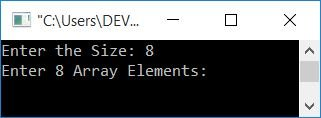Enter all 8 array elements, namely 1, 2, 3, 4, 2, 5, and 2, and press the ENTER key.Here is the output you will see: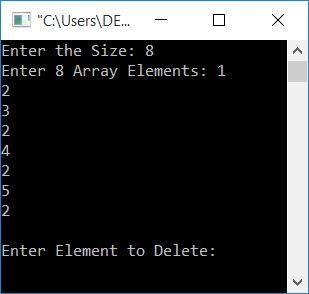Now finally enter 2 as an element to remove or delete and press the ENTER key to delete 2 from the list and print the new array as shown in the snapshot given below: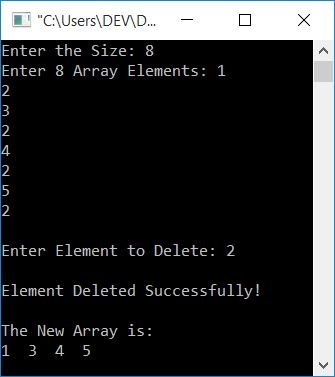### Using a function, delete an element from an array

This program is the complete version of deleting an element from an array in C++. It produces the output based on the input. At runtime, it also handles invalid user input. This program uses the user-defined function delElem().

The function delElem() takes three arguments. The first argument is array, the second is size, and the third is the element that has to be deleted. To learn more about function in C++, you can refer to its separate tutorial.

```#include<iostream>
using namespace std;
int delElem(int [], int, int);
int main()
{
int arr, tot, i, elem, chk;
cout<<"Enter the Size: ";
cin>>tot;
if(tot>0)
{
cout<<"Enter "<<tot<<" Array Elements: ";
for(i=0; i<tot; i++)
cin>>arr[i];
cout<<"\nEnter Element to Delete: ";
cin>>elem;
chk = delElem(arr, tot, elem);
if(chk==101)
cout<<"\nElement doesn't found in the Array!";
else if(chk==102)
{
cout<<"\nElement Deleted Successfully!";
cout<<"\n\nThe New Array is:\n";
cout<<"Empty!";
}
else
{
cout<<"\nElement Deleted Successfully!";
cout<<"\n\nThe New Array is:\n";
for(i=0; i<chk; i++)
cout<<arr[i]<<"  ";
}
}
else
cout<<"\nInvalid Input!";
cout<<endl;
return 0;
}
int delElem(int arr[], int tot, int elem)
{
int i, j, found=0;
for(i=0; i<tot; i++)
{
if(arr[i]==elem)
{
for(j=i; j<(tot-1); j++)
arr[j] = arr[j+1];
found++;
i--;
tot--;
}
}
if(found==0)
return 101;
else if(tot==0)
return 102;
else
}```

Here is its sample run with user input: 5 as size, 1, 2, 3, 2, 4 as 5 array elements, and 2 as an element to delete:Here is another sample run with user input: 5 as size, 2, 2, 2, 2, 2 as 5 array elements, and 2 as an element to delete: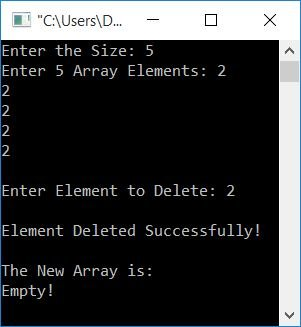Here is another sample run with user input: 5 as size, 6, 7, 8, 9, 10 as 5 array elements, and 3 as an element to delete: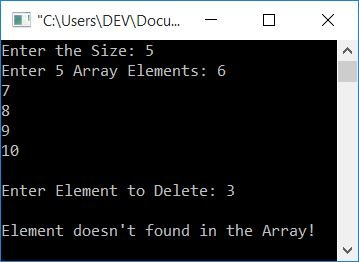And if the user enters input, that is not a valid number for the above program. For example, a, -4, 0, # etc. The program will then display an error message such as "Invalid Input!"

#### Same Program in Other Languages

C++ Online Test

« Previous Program Next Program »